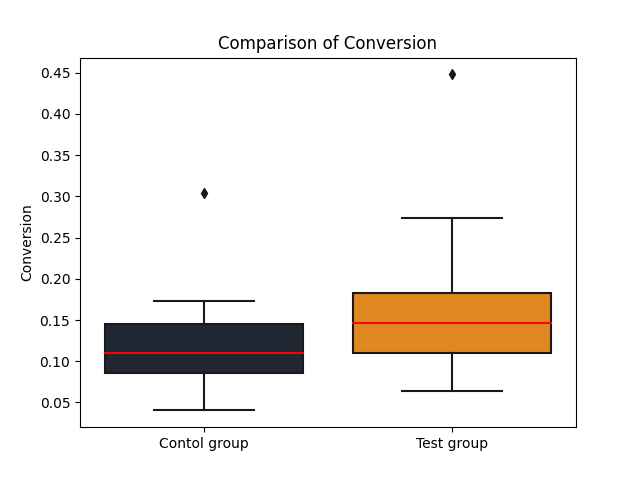Course Content

The Art of A/B Testing

##The First U-Test

The U-test, also known as the Mann-Whitney test, is a nonparametric statistical test used for comparing two independent samples. It is used when the data do not meet the assumptions of parametric tests, such as when the data distribution is non-normal or when the data are measured on an ordinal scale.

The U-test assesses whether there is a statistically significant difference between the medians of the two groups. It does not rely on any prior assumptions about the data distribution and is relatively robust to outliers or unusual values. Let's see how the U-test performs for our samples. Let's formulate the hypotheses:

H₀: The medians of the `'Conversion'` metric in the control and test groups are equal.

Hₐ: The medians of the `'Conversion'` metric differ between the control and test groups.The obtained statistical value and the low p-value indicate a statistically significant difference between the medians of the `'Conversion'` metric in the control and test groups. We have sufficient evidence to reject the null hypothesis and accept the alternative hypothesis that the medians differ.

This means that there are statistically significant differences in the `'Conversion'` metric between the control and test groups.Based on the box plot, we can conclude that the median of the `'Conversion'` metric in the test group is higher than the median in the control group.

Now it's your turn to conduct the U-test.